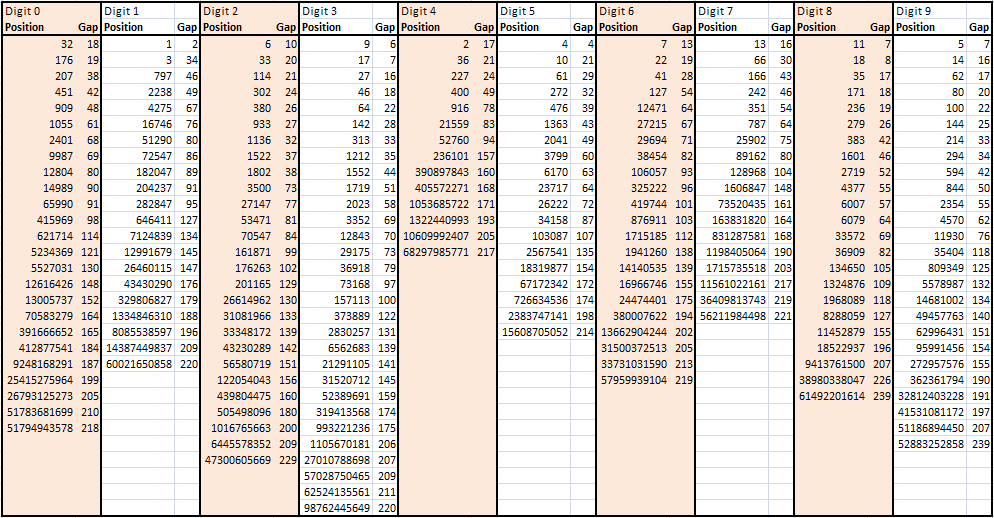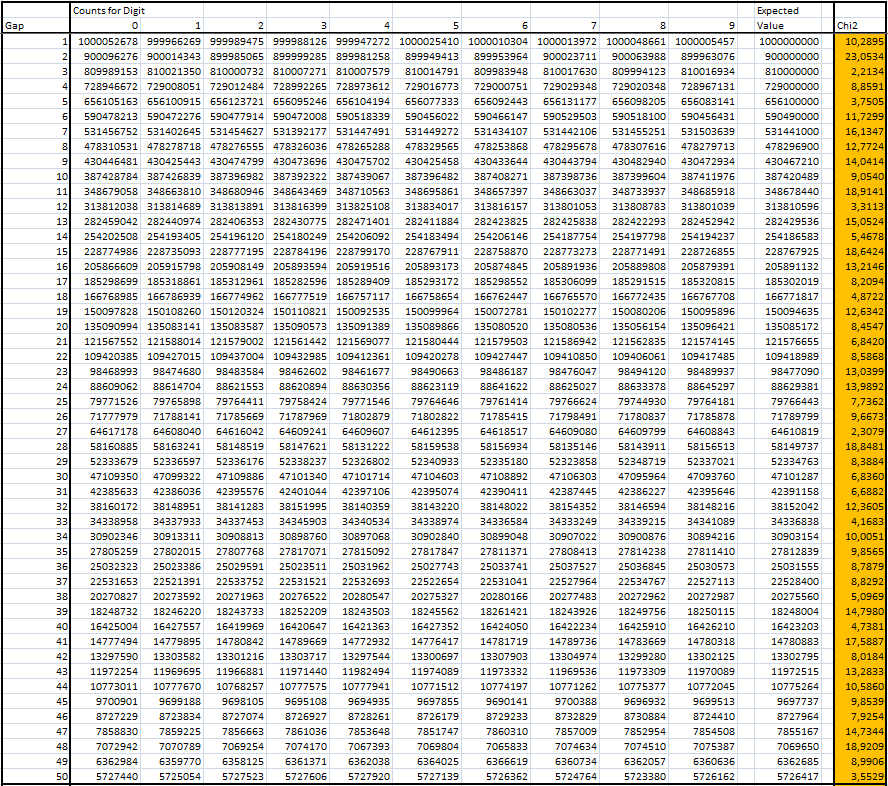# What’s the (longest) distance between two identically digits?

## GENERAL CONSIDERATIONS / EXPECTED VALUES

The Gap Test measures the distance between two successive appearances of the same digit and divides the whole sequence into separate chains of various length.
Here is the example for digit "1":

1 4 >> gap = 2
1 5 9 2 6 5 3 5 8 9 7 9 3 2 3 8 4 6 2 6 4 3 3 8 3 2 7 9 5 0 2 8 8 4 >> gap = 34
1 9 7 >> gap = 3
1 6 9 3 9 9 3 7 5 >> gap = 9
and so on ...

A gap of length 1 is simply a pair of identically digits. In general the probability to find a chain of length L with nonrepeating starting digit is

w = 1/10 x (9/10)L-1

From there we expect an average chainlength of L = 10. So running the test on N digits we will get approximativly N/10 chains. We can estimate the maximum chain length from N ~ 1/w = 10*(10/9)L-1 which gives a estimated value Lmax = 220.

## Result: Maximum Gap for digits 0-9## Result: Increase of the longest Gap for digits 0-9 while stepping through Pi-sequence## Result: Number of Different Gap Lengths for any Digit 0 to 9

Critical Value of Chi2-Distribution for Rows on 99,5% Level (f=9): 23,589
Critical Value of Chi2-Distribution for Columns on 99,5% Level (f=99): 138,987..........